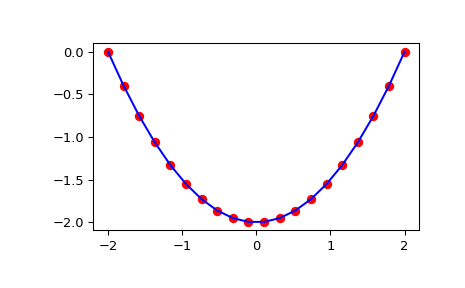# scipy.integrate.cumulative_trapezoid¶

scipy.integrate.cumulative_trapezoid(y, x=None, dx=1.0, axis=- 1, initial=None)[source]

Cumulatively integrate y(x) using the composite trapezoidal rule.

Parameters
yarray_like

Values to integrate.

xarray_like, optional

The coordinate to integrate along. If None (default), use spacing dx between consecutive elements in y.

dxfloat, optional

Spacing between elements of y. Only used if x is None.

axisint, optional

Specifies the axis to cumulate. Default is -1 (last axis).

initialscalar, optional

If given, insert this value at the beginning of the returned result. Typically this value should be 0. Default is None, which means no value at `x` is returned and res has one element less than y along the axis of integration.

Returns
resndarray

The result of cumulative integration of y along axis. If initial is None, the shape is such that the axis of integration has one less value than y. If initial is given, the shape is equal to that of y.

`numpy.cumsum`, `numpy.cumprod`
`quad`

`romberg`

`quadrature`

`fixed_quad`

`dblquad`

double integrals

`tplquad`

triple integrals

`romb`

integrators for sampled data

`ode`

ODE integrators

`odeint`

ODE integrators

Examples

```>>> from scipy import integrate
>>> import matplotlib.pyplot as plt
```
```>>> x = np.linspace(-2, 2, num=20)
>>> y = x
>>> y_int = integrate.cumulative_trapezoid(y, x, initial=0)
>>> plt.plot(x, y_int, 'ro', x, y + 0.5 * x**2, 'b-')
>>> plt.show()
```